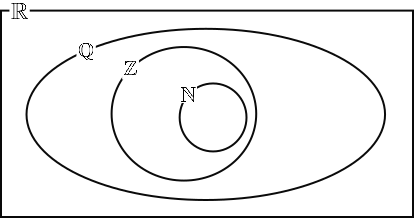# Exam-Style Questions on Number

## Problems on Number adapted from questions set in previous Mathematics exams.

### 1.

GCSE Higher

Without using a calculator:

(a) Express 68 as the product of its prime factors.

(c) Multiply 13.06 by 2.1

### 2.

GCSE Higher

The number, $$N$$, can be written as the product of prime factors in index form as:

$$N = 3 × 5^3 × x^4$$

Work out $$5N^2$$ as a product of prime factors in index form giving your answer in terms of $$x$$.

### 3.

GCSE Higher

The difference between the areas of the two squares is 51 cm2.

When measured in cm the lengths of the sides of the squares are integers and both less than 20cm.

Find the lengths of the sides of the two squares.

### 4.

IB Studies

This Venn diagram shows the relationship between the sets of numbers

$$\mathbb N, \mathbb Z, \mathbb Q \text{ and } \mathbb R$$Write down the following numbers in the appropriate place in the Venn diagram.

(a) $$5$$

(b) $$\frac23$$

(c) $$\pi$$

(d) $$0.91$$

(e) $$\sqrt 7$$

(f) $$-0.75$$

### 5.

GCSE Higher

This expression can be used to generate a sequence of numbers.

$$n^2+n + 5$$

(a) Work out the first three terms of this sequence.

(b) What is the smallest value of $$n$$ that produces a term of the sequence that is not a prime number?

(c) Is it true that odd square numbers have exactly three factors? Explain and justify your answer.

(d) Seymour is thinking of a number.

• It is a common factor of 144 and 192.
• It is a common multiple of 6 and 8.
• It is less than 100.

Find the two possible numbers that Seymour could be thinking of.

### 6.

GCSE Higher

(a) Write this list of numbers in order, smallest first.

$$\sqrt{47}, \frac{22}{3}, 3.5^2, 8.64$$

(b) Write $$(1 + \sqrt5)^2$$ in the form $$a + b\sqrt5$$ .

The exam-style questions appearing on this site are based on those set in previous examinations (or sample assessment papers for future examinations) by the major examination boards. The wording, diagrams and figures used in these questions have been changed from the originals so that students can have fresh, relevant problem solving practice even if they have previously worked through the related exam paper.

The solutions to the questions on this website are only available to those who have a Transum Subscription.

Exam-Style Questions Main Page

Search for exam-style questions containing a particular word or phrase:

To search the entire Transum website use the search box in the grey area below.

Kausar Begum Khan, Kenya

Friday, January 26, 2018

"This is a fabulous website! I cannot thank you enough."

Tuesday, November 6, 2018

"Transum is so resourceful. The resources are so child-friendly that my students love its usage.It has a wide range of topics and caters to most of my needs. 'Thankyou" will be too small a word to express my gratitude to the content developers of this site."

Ahmed Jaja, Ghana

Thursday, November 22, 2018

"It was very useful for me.I recommend it to every math student.Thank you."

Tuesday, February 5, 2019

"A very great site.Nice questions."

Yuli, Woodmansterne

Tuesday, April 2, 2019

"This website is amazing it helps me with my maths to get good marks in my upcoming test. Thanks."

Do you have any comments? It is always useful to receive feedback and helps make this free resource even more useful for those learning Mathematics anywhere in the world. Click here to enter your comments.For All: